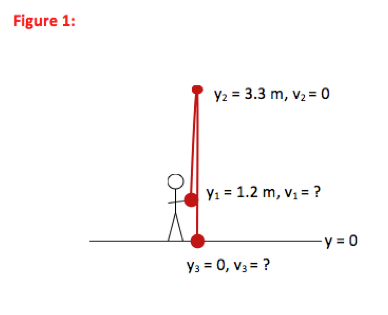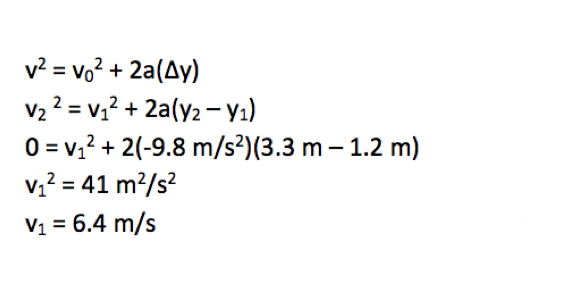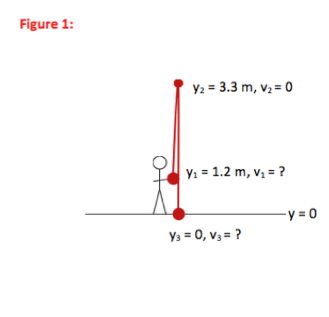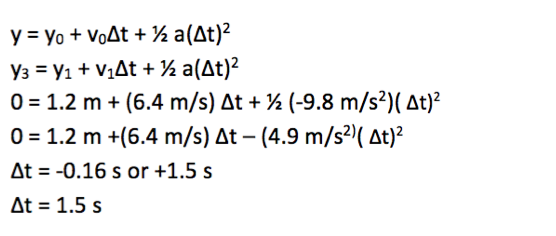# University of Wisconsin Green Bay

You want to throw a ball straight up into the air so that it reaches a height of 3.3 m above the ground.
a) If the ball leaves your hand at a height of 1.2 m above the ground, how fast do you need to throw it?
b) How long does it take the ball to hit the ground? You may ignore any effects of air resistance throughout this problem.

• In this problem, you are asked to describe the motion (how fast, how far, how long) of the ball. Whenever you are asked to describe the motion of an object without worrying about the cause of that motion, you have a kinematics problem.

••There are three key kinematic equations. If you carefully select the equation which most directly describes the situation in your problem, you will not only solve the problem in fewer steps but also understand it better. The three equations, written for motion in the y-direction, are:

1. y = y0 + v0Δt + ½ a(Δt)2 (relates position and time)
2. v = v0 + aΔt (relates velocity and time)
3. v2 = v02 + 2a(Δy) (relates velocity and position)

a) The initial velocity of the ball is a variable in all three equations, so this question drives home the point that there is more to selecting the appropriate relationship than just noting what value is requested in the problem. In this case, you want to find the starting velocity that gives a maximum height of 3.3 m. Maximum height is the position at which y-velocity is zero. The question relates position and velocity, so you want to use equation 3.

b) You are asked how long (time) it takes the ball to reach the ground (position), so you want to use equation 1.

• Step 1:The velocity when the ball left your hand (Point 1) is the information that was requested in part a) of the problem.

--------------------------------------------------------------------------------------------

Step 2:Part b) of the problem asked for the amount of time the ball was in the air (between Point 1 and Point 3.) No further mathematical solution is required.

•v22 = v12 + 2a(y2 – y1)
0 = v12 + 2(-9.8 m/s2)(3.3 m – 1.2 m)
v1 = 6.4 m/s

In this problem, you were first asked to find how fast you needed to throw the ball. In other words, you were asked to find the initial velocity of the ball. Because all equations include initial velocity, you could not use that as a criterion to select the best formula. However, you know that the ball reaches a maximum height (vy = 0 at the top of the motion) of 3.3 m, so the best relation to select is the one that relates position and velocity. The speed of 6.4 m/s is about 14 mph, so is a reasonable answer.

y3 = y1 + v1Δt + ½ a(Δt)2
0 = 1.2 m + (6.4 m/s) Δt + ½ (-9.8 m/s2)( Δt)2
Δt = -0.16 s or +1.5 s
Δt = 1.5 s

In part b), you were asked to find how long (time) it took the ball to reach the ground (position) and so the position-time equation was used between the initial and final locations. Because the position-time equation includes a ( Δt)2 term, it will always give two answers for time. The solution that makes physical sense is the positive time, or 1.5 s after the ball leaves your hand.